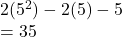## If f(x) = 2x² – 4x and g(x) = 5 – 2x, evaluate fx) – g(x) for x = 5. A 15 B 5 C 35 D. 20 ET

Question

If f(x) = 2x² – 4x and g(x) = 5 – 2x, evaluate fx) – g(x) for x = 5.
A 15
B 5
C 35
D. 20
ET

in progress 0
6 months 2021-09-03T18:27:16+00:00 1 Answers 2 views 0

## Answers ( )

35

Step-by-step explanation:

2x² – 4x- (5 – 2x)

2x² – 4x – 5 + 2x

2x²-2x – 5 = f(x) – g(x)

Now use 5